# Self-Organized Criticality in the Universe

### Self-organized criticality is a theoretical concept that describes the statistics of nonlinear processes. It is a fundamental principle that is common to many nonlinear dissipative systems in the universe. Due to its universality and ubiquity, SOC is essentially a law of nature.

August 15, 2011

Markus J. Aschwanden is a Solar Physicist at the Solar and Astrophysics Laboratory of the Lockheed Martin, Advanced Technology Center in Palo Alto, CA. He has authored many books in Solar Physics, the most recent is the Self-Organized Criticality in Astrophysics – The Statistics of Nonlinear Processes in the Universe.

Little children like to play with sand piles, piling up cone-like mountains and sprinkling handfuls of sand over the steep slopes to watch small and large sand avalanches rolling downhill. These children probably never realize that they conduct experimental tests of a physical theory that is now called “self-organized criticality”, abbreviated as SOC. Self-organized criticality is a theoretical concept that describes the statistics of nonlinear processes. It is a fundamental principle that is common to many nonlinear dissipative systems in the universe. Due to its universality and ubiquity, SOC is essentially a law of nature.

The SOC concept was introduced in a seminal paper by Bak, Tang, and Wiesenfeld in 1987. The principle can be best understood in terms of avalanches on a sandpile (Figure 1). Suppose we start from scratch and build a sand pile by randomly adding sand, a grain at a time. The pile will grow, and the slope will increase. Eventually, the slope will reach a critical value (called the “angle of repose”, which is typically ~ 35\deg for dry sand); if more sand is added it will slide off. Alternatively, if we start from a situation where the pile is too steep, the pile will collapse until it reaches the critical slope again. So, the critical slope is self-organizing in the sense that it adjusts always near to the critical value. The input can be a steady rate of dropped sand grains, but the output are avalanches of arbitrary sizes, either small or large, independent of the input, randomly occurring. The reason how large avalanches result is a property of chain reactions, which grow in a multiplicative way, rather than in a linear fashion. However, the nonlinear system needs to be in a critical state. If the sandpile does not has the critical slope (right panel in Figure 1), such as a flat sand beach (left panel in Figure 1), nothing happens in such a stable system.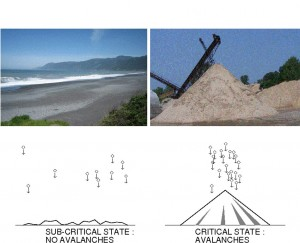Figure 1. A flat landscape produces no avalanche events (bottom left panel), such as the flat sand beach in northern California (top left panel), while randomly dripping sand onto a sandpile produces a state of self-organized criticality where avalanches occur (bottom right panel), such as with the conveyor belt of the Indian River Enterprises (top right panel).

If you roll two six-sided dices, you will find that a sum of 2 (=1+1) is as rare as a sum of 12 (=6+6), while the value of 7 is most likely, because it has most of the possible combinations, such as 1+6=7, 2+5=7, 3+4=7, 4+3=7, 5+2=7, 6+1=7). So, multiple dices produce random numbers, but the distribution of possible outcomes forms a binomial distribution, which turns into a normal or Gaussian distribution for a large number of dices. Sand avalanches have a completely different statistics: the smallest avalanches are most common, while very large avalanches are very rare. The same is true for earthquakes, hurricanes, tornados, or forest fires. It has to do with the way how many next-neighbor elements are involved when an avalanche starts. Avalanches in a critical system propagate like a chain reaction, where the number of elements grows in a multiplicative manner: one sand grain brings two other grains into rolling, the two grains hit four, the four grains hit eight, and so forth. We call such a situation a nonlinear system, because processes can grow in a nonlinear way, such as an exponential function in a multiplicative chain reaction.

The difference between a linear and a nonlinear system is shown in Figure 2: A hydropower plant can be described as a linear system, since the produced electric energy is proportional (or linear) to the water input that flows out of a dam into the pipeline of the power plant (Figure 2, top). A snow avalanche, however, is a nonlinear system, because the output (in form of a rolling avalanche) is a nonlinear function with respect to the input (which can be a slight temperature change in the snow).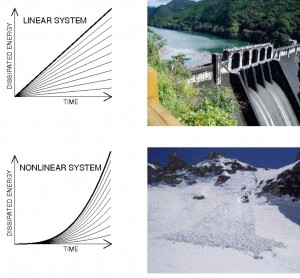Figure 2. The output in a linear system grows linearly with time, for a constant input rate (top left panel), while the output is highly unpredictable and uncorrelated with the input rate in a nonlinear system (bottom left panel). A practical example of a linear system is a hydroelectric plant, where the produced electric energy is proportional to the water input, as depicted with the water-storage dam at Yaotsu, Gifu, Japan (top right panel). A classical example of a (dissipative) nonlinear system is a snow avalanche, as shown in the large wet-snow avalanche at Deadman Canyon in the Sierra Nevada range (bottom right panel).

The principle of SOC has been found to operate not only in sandpiles, which have been subjected to quantitative experiments in laboratories, but was also found in many other processes in our daily life, as well as in the rest of the universe, essentially wherever multiplicative chain reactions can occur in systems in a critical state. The most popular ones are known in geophysics, such as earthquakes, landslides, and forest fires. A statistics of earthquakes is shown in Figure 3. Geologists plot the logarithm of the number of earthquakes, log(N), versus the logarithm of the size, log(S), which they call a “log N-log S plot”. For SOC systems, the resulting distribution is a straight line, which is mathematically called a “powerlaw function”. From such a distribution as shown in Figure 3, the likelyhood of an earthquake can be read off: The probability of an earthquake with magnitude 7 is about 0.03 (or 3%) per year, so it happens about every 30 years. If we look at a 10 times larger event, with magnitude 8, the probability is exactly 100 times lower, because the distribution has a negative powerlaw slope of -2, which is the 10-logarithm of 1/100.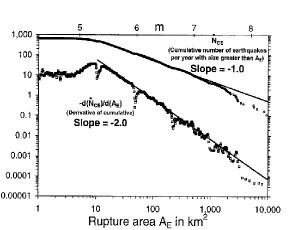Figure 3. World-wide cumulative and differential frequency distribution of earthquakes per year as a function of the rupture area (in units of square kilometers), based on the Harvard Centroid-Moment Tensor Data Base (1997) for the years 1997-1994. The conversion into equivalent Gutenberg-Richter magnitudes \$m\$ is indicated on the top axis.

Even more interesting, many statistics in magnetospheric physics (auroral blobs), solar physics (flares), and in stellar physics (flares, cataclysmic variables, accretion disks, black holes, pulsar glitches, gamma ray bursts) have also shown powerlaw-like log N-log-S plots, which are also called “occurrence frequency distributions”, another name for probability or likelyhood. Solar flares are huge magnetic instabilities in the solar atmosphere, where magnetic field changes induce strong currents and lead to plasma heating to several tens of million degrees Kelvin. The free magnetic energy accelerates particles (electrons and ions), which slam into the solar chromosphere and heat the plasma there, which streams up into the upper corona along the magnetic field lines and shows us beautiful arcades of filled magnetic loops, as shown in Figure 4. However, these arcades of hot plasma (a gas that is so hot that electrons and ions become separated inside an atom) can only be observed in hard X-rays, soft X-rays, and extreme-ultraviolet, wavelengths that are filtered out by the Earth atmosphere and thus have to be observed with spacecraft. The magnetic or thermal energy of a solar flare as shown in Figure 4 can be calculated from the brightness and this way we can determine some magnitude like in earthquakes. An occurrence frequency distribution of a large set of solar flares is shown in Figure 5, which shows a perfect powerlaw and is likely to be the result of a self-organized critical system in the solar corona. Apparently, little disturbances of the magnetic field can propagate in a multiplicative chain reaction of so-called “magnetic reconnection” events, in an analog way as in earthquakes or in forest fires.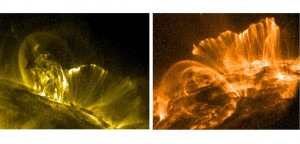Figure 4. Solar flares (observed in the extreme ultra-violet wavelength of 171 \AA ) with the NASA spacecraft TRACE: The flare of 2001 Apr 15 exhibits an erupting filament in the foreground and a rising postflare arcade behind near the limb (left panel), while the 2000 Nov 9 flare displays the 3-D geometry of the double-ribbon postflare arcade (right panel).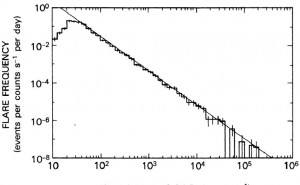Figure 5. Occurrence frequency distribution of the peak count rate of over 6000 hard X-ray flares with photon energies above 25 keV, recorded with the Hard X-Ray Burst Spectrometer (HXRBS) onboard NASA’s Solar Maximum Mission (SMM) during 1980-1985. The distribution follows a powerlaw with a slope of ~ 1.8 over 4 orders of magnitude.

“How can the universe start with a few types of elementary particles at the big bang, and end up with life, history, economics, and literature? The question is screaming out to be answered but it is seldom even asked. Why did the big bang not form a simple gas of particles, or condense into one big crystal ?”

This is how Per Bak, the father of the theory on self-organized criticality, phrased the problem to understand the complexity we see in our world, in his popular book “How Nature Works” that was published in 1996. Self-organized criticality seems also to occur in our daily life. If we look at the size of cities, as shown in the satellite picture of North America at night (Figure 6), we find that the distribution of city sizes (by the number of population as determined from the census of 276 metropolitan areas in the USA in 2000), we find again a powerlaw-like distribution (right panel in Figure 6). Obviously, population growth in cities occurs also in a multiplicative manner. Similar statistics is found for stock market fluctuations, for wheat or cotton prices, for lottery wins, for traffic jams, for word usage, as well as for internet traffic.

Let us think about a specific example. If one student decides to learn 10 new words in a foreign language every day, he will find that the amount of literature he can understand will grow exponentially, rather than linearly with the number of new learned words, because sentences are composed of many possible combinations of the same words. If the student continues to learn a language over years by learning 10 new words every day, suddenly he will understand most complex information and insights. Nonlinear phenomena, leading to life, history, economics, and literature, developed in the same way, which makes our universe to a fascinating place of unpredictable complexity.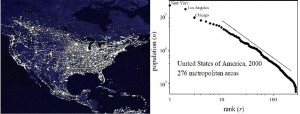Figure 6. Left: Satellite picture of North America at night, taken from the orbiting International Space Station (NASA). The sizes of the light dots scale with the sizes of cities. Right: Zipf rank plot for 276 metropolitan areas in the United States, after results of the census in 2000.